### Home > PC > Chapter 1 > Lesson 1.1.1 > Problem1-5

1-5.
1. Sketch the graph of each function below. Record the name of each function next to its sketch. You may notice that each of these graphs is a parent graph. 1-5 HW eTool (Desmos). Homework Help ✎

1. y = x2

2. y = x3

3. y =4. y = 2x

5. y =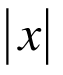6. y =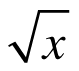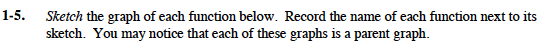Use the eTool provided below to help you graph the functions you may be unfamiliar with.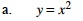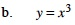cubic function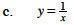hyperbola or inverse function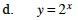exponential function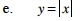absolute value function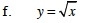square root function